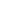Original research

# Heart plaque detection with improved accuracy using logistic regression and comparing with least squares support vector machine

## Abstract

Aim: The major goal of this study is to compare the effectiveness of the Logistic Regression classifier with the Least Squares Support Vector Machine classifier in detecting plaque in the heart with high accuracy. Materials and Methods: In this work, the Logistic Regression and least squares Support Vector Machine methods are compared. There were a total of 20 samples in the Kaggle dataset on Heart Plaque disease. To calculate sample G power of 0.08 with 95% confidence interval, Clincalc is utilized. There are two groups in the training dataset (n = 489 (70 percent)) and the test dataset (n = 277 (30 percent)). Results: The accuracy of both the Logistic Regression and Least Squares Support Vector Machine algorithms is evaluated. The Least Squares Support Vector Machine approach was only 67.3 % accurate, while the Logistic Regression method was 96 % accurate. Since p(2-tailed) < 0.05, in SPSS statistical analysis, a significant difference exists between the two groups. Conclusion: The Logistic Regression algorithm is significantly better than Least Squares Support Vector Machine algorithm in this study in detecting cardiac plaque disease in the dataset.

## Imprint

Vankamaddi Sunil Kumar, K Vidhya. Heart Plaque detection with improved accuracy using Logistic Regression and comparing with Least Squares Support Vector Machine . Cardiometry; Issue 25; December 2022; p.1600-1604; DOI: 10.18137/cardiometry.2022.25.16001604; Available from: https://www.cardiometry.net/issues/no25-december-2022/logistic-regression-comparing

## Keywords

Heart Plaque,   Novel texture feature,   Logistic Regression algorithm,   Least Squares Support Vector Machine,   Prediction,   Machine learningCurrent issueCardiometry's libraryFounders of Cardiometry